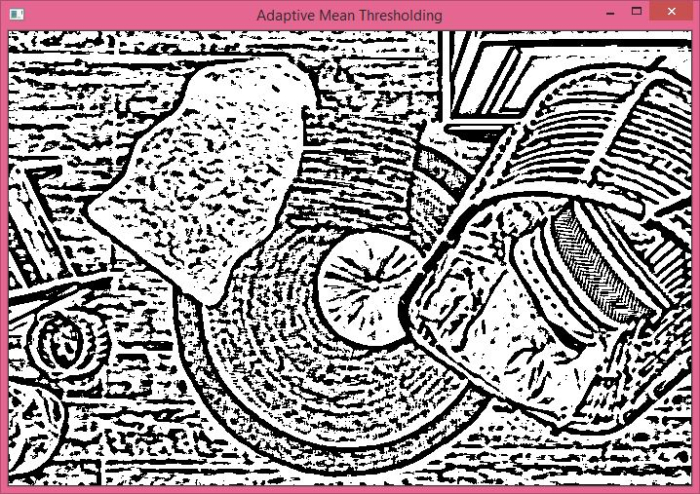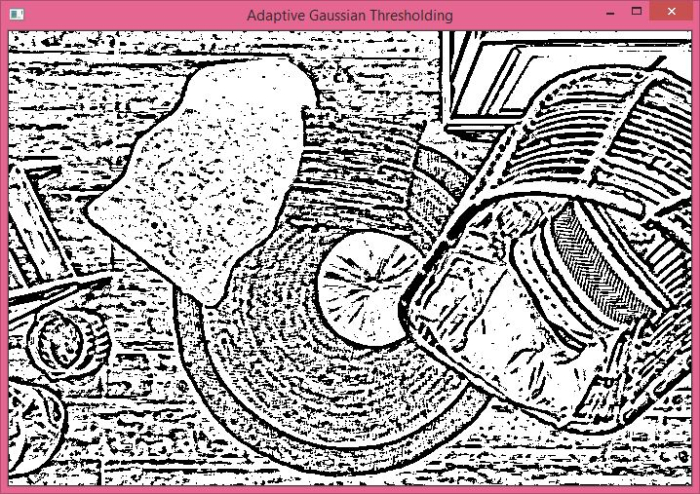# How to perform adaptive mean and gaussian thresholding of an image using Python OpenCV?

Adaptive thresholding is a kind of thresholding technique. There are other types of thresholding techniques such as simple thresholding that uses a global threshold value. But using a global threshold value is not a good idea for an image having different lighting conditions in different areas.

Adaptive thresholding calculates the threshold value for a small region in the image. So we have different threshold values for different regions in the image which gives better results in comparison to the simple thresholding technique.

There are three special parameters: adaptive_method, block_size and const. See the syntax given below.

## Syntax

cv2.adaptiveThreshold(img, max_val, adaptive_method, thresh_type, block_size, const)


## Parameters

• img − Input gray scale image. It's a numpy.ndarray.

• max_valu − Maximum value to be assigned to a pixel.

• adaptive_method − It decides how thresholding value is calculated. There are two different adaptive thresholding methods −

• cv2.ADAPTIVE_THRESH_MEAN_C − In this method, threshold value is the mean of neighborhood area.

• cv2.ADAPTIVE_THRESH_GAUSSIAN_C − In this method, threshold value is the weighted sum of neighborhood values where weights are a gaussian window.

• thresh_type − The type of thresholding to be applied.

• block_size − Decides the size of the neighborhood area.

• const − A constant subtracted from the mean or weighted mean calculated.

Output − It returns the threshold

Let's understand the Adaptive Mean thresholding and Adaptive Gaussian thresholding with the help of a couple of Python examples.

## Input Image:

We will use this image as the input file in the following examples.## Example 1: Adaptive Mean Thresholding

In this program, we apply adaptive thresholding on the input image using cv2.ADAPTIVE_THRESH_MEAN_C as an adaptive method. We use block_size=11 and const=2. We use Binary thresholding as thresh_type.

import cv2

# Read the input RGB image as a Gray Scale image

# apply median blur
img = cv2.medianBlur(img,5)

# display the output image
cv2.waitKey(0)
cv2.destroyAllWindows()


## Output

When you run the above program, it will produce the following output window −The above output shows the image after applying Adaptive Mean Thresholding.

## Example 2: Adaptive Gaussian Thresholding

In this program, we apply adaptive thresholding on the input image using cv2.ADAPTIVE_THRESH_GAUSSIAN_C as an adaptive method.

We use block_size=11 and const=2. We use Binary thresholding as thresh_type.

import cv2
# Read the input RGB image as a Gray Scale image

# apply median blur
img = cv2.medianBlur(img,5)

# display the output image
cv2.waitKey(0)
cv2.destroyAllWindows()


## Output

When you run the above program, it will produce the below output.The above output shows the image after applying Adaptive Gaussian Thresholding.

Updated on: 27-Sep-2022

1K+ Views